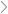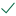Questions & AnswersStatistics and ProbabilityGraph the following non-linear system of equations and identify the...

QuestionAnswered step-by-step

# Graph the following non-linear system of equations and identify the...

Graph the following non-linear system of equations and identify the two solutions.

(Remember that for a system of equations to a linear system, all the equations would have to be linear.

If even one function is not linear, the whole system is called non-linear)

y=x2+6x+7 = 2+6 +7

y=x+3 = +3

What type of function is the equation y=x2+6x+7�=�2+6�+7?

What type of function is the equation y=x+3�=�+3 ?

Use your graph below to identify the two solutions to this non-linear system of equations.

Note: All solutions to this problem are whole numbers. None of the answers are decimals or fractions.

List the solution, (x,y), with the smallest x value below.

x=�=

y= =

List the solution, (x,y), with the largest x value below.

x=�=

y= =Solved by verified expert

• sectetur

• sectet

• sectet
• sectet

• sectet
• secte
sectetur adipiscing elit. Nam lacinia pul10,000 step-by-step explanations

## Step-by-step explanation

sectetur adipiscing elit. Nam lacinia pulvinar tortor nec facilisis. Pellentesque dapibus e

sectetur adipiscing elit. Nam lacinia pulv

sectetur adipiscing elit. Nam lacinia pulvinar tortor n

sectetur adipiscing elit. Nam lacinia pulvinar to

sectet

sectetur